## Length Converter calculator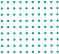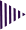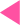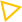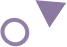=
Best length conversion calculator

# Online length conversion calculator

The term length refers to the distance between two points. The International System of Quantities recognizes length as a quantity with a dimension of distance.
Total calculators capable to present one of the best free online calculator for you. The process of changing lengths between different units is called length conversion. For example, kilometers can be converted to meters, Miles can be converted to meters, yards can be changed to feet, and so on. The conversion of length between different scales is necessary for matching measurements.
1 mile = 1760 yards or 1609 meters
1 rod = 5.50 yards or 5.029 meters
1 yard = 3 feet or 0.9144 meters
1 foot = 12 inches or 0.3048 meters
1 Inch = 0.083 foot or 0.0254 meters

### Length Conversion Units Definitions

• Meter converter definition:-

Metres are SI units of distance. Meters are devices that measure and may record a magnetic or electrical quantity, such as voltage or current. Metric lengths are measured in meters for example. 1 meter = 100 centimeters

• Milimeter converter definition:-

Millimeters are metric units of length that are equal to one thousandth of a metre, which is the SI base unit of length. As an example, a metre contains 1000 millimetres, and a centimetre contains 10 millimetres.

• Centimeter converter definition:-

-In metric measurements, a centimeter is used for measuring lengths of objects. One centimeter is half the size of an inch, which means that two-and-a-half centimeters make one inch. A measurement of length. for example One metre equals 100 centimeters

• Decimeter converter definition:-

This unit is used to measure length. 1 metre equals 10 decimetres, one decimeter is 1/10 of a meter in length. for example A decimeter is about 4 inches in length.
A metric system of measurement is 10 decimeters = 1 meter, 100 square meters. A meter is equal to 1 are ; 10 meters are equal to 1 dekameter ; 100 ares are equal to 1 hectare ; 10 dekameters are equal to first hectometer.